# ML Aggarwal Solutions for Class 7 Maths Chapter 16 Perimeter and Area

ML Aggarwal Solutions for Class 7 Maths Chapter 16 Perimeter and Area provide information on determining the area and perimeter of the given figure. The solutions are briefed step by step, as per the students intelligence quotient. Regular practice of these solutions speed up the capacity of solving problems effortlessly among students. In order to secure more marks, students can refer to ML Aggarwal Solutions for Class 7 Maths Chapter 16 Perimeter and Area PDF, from the links which are presented below.

Chapter 16 deals with the study of finding the perimeter and area of a particular figure. The various problems present in the chapter improves students’ knowledge about the concepts within a short period. This also helps them to face the examination fearlessly.

## ML Aggarwal Solutions for Class 7 Maths Chapter 16 Perimeter and Area Download PDF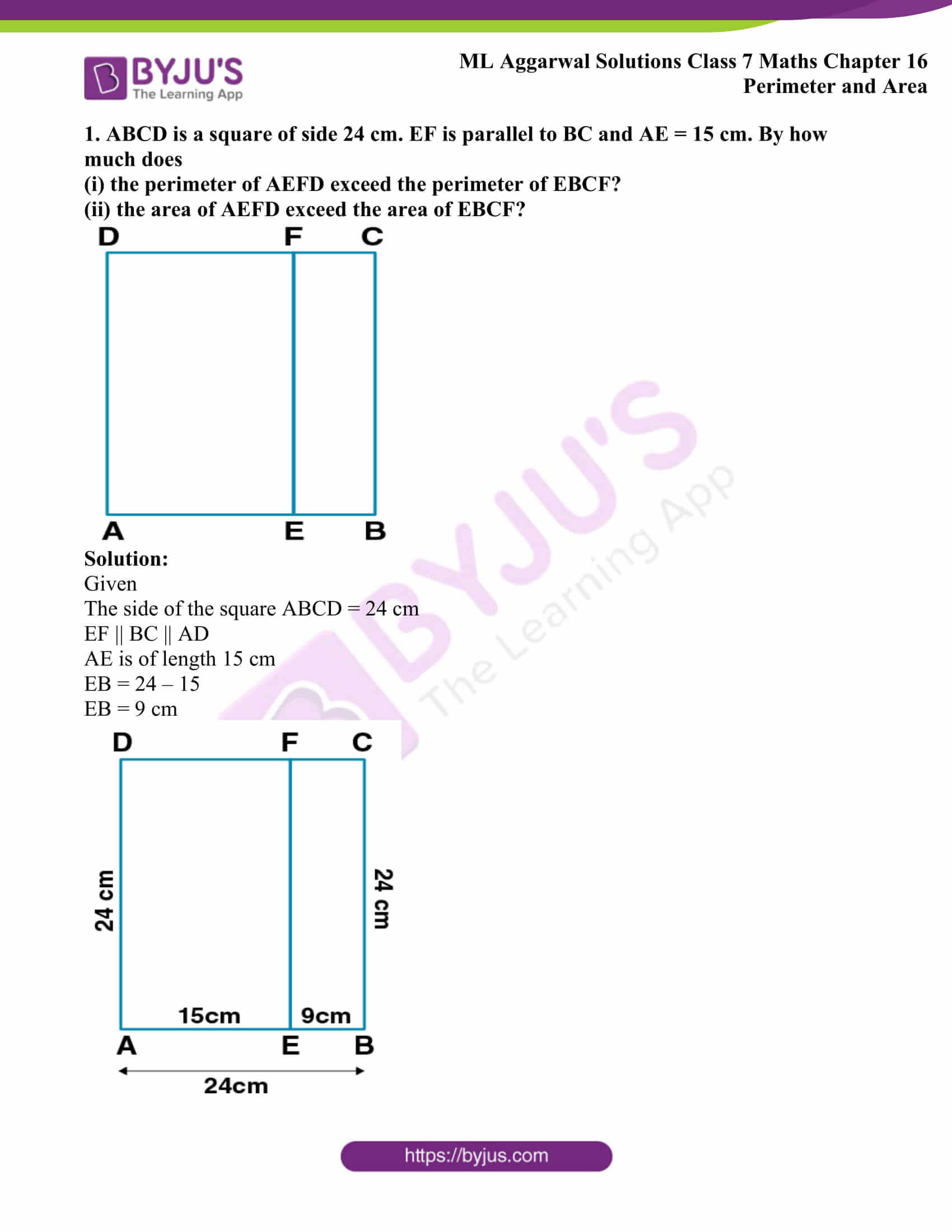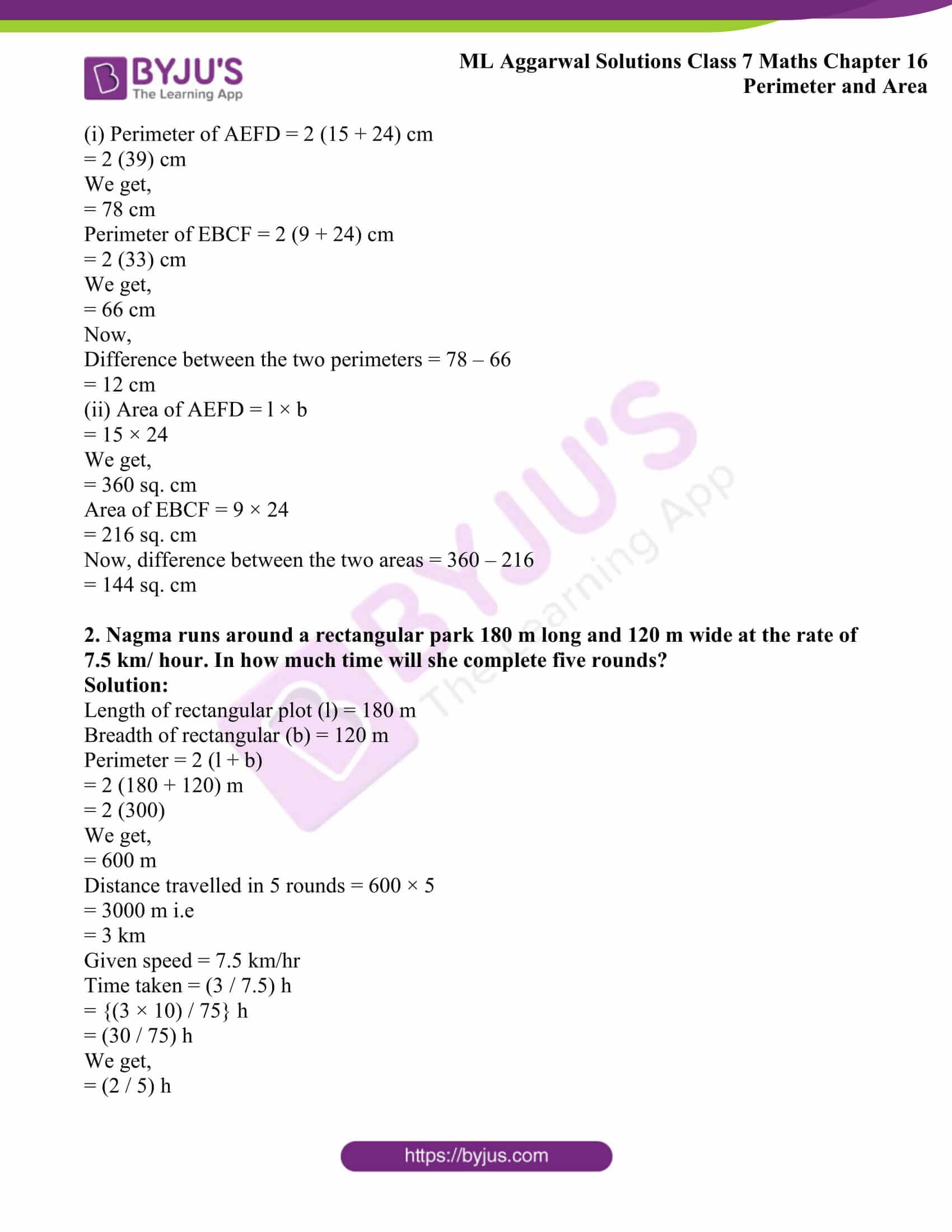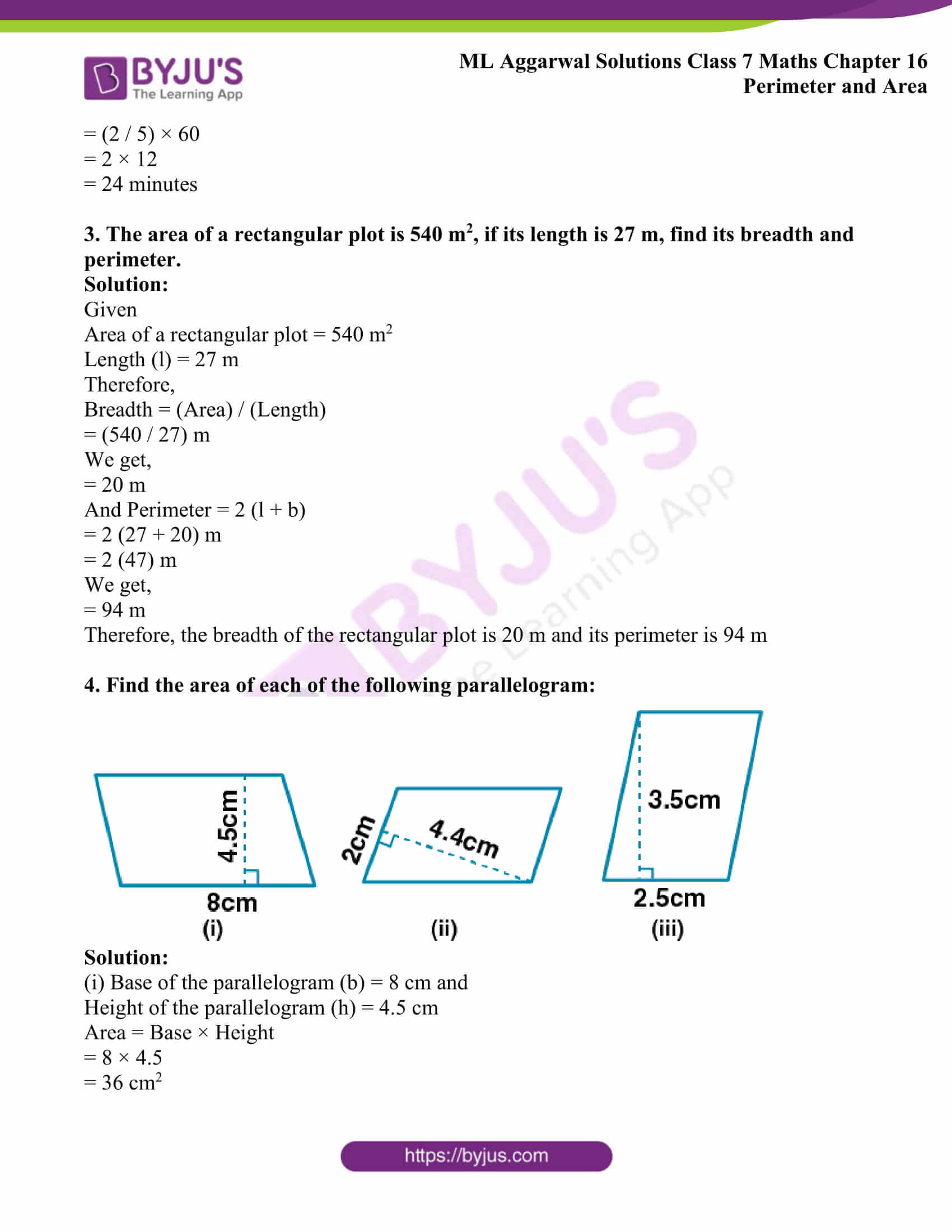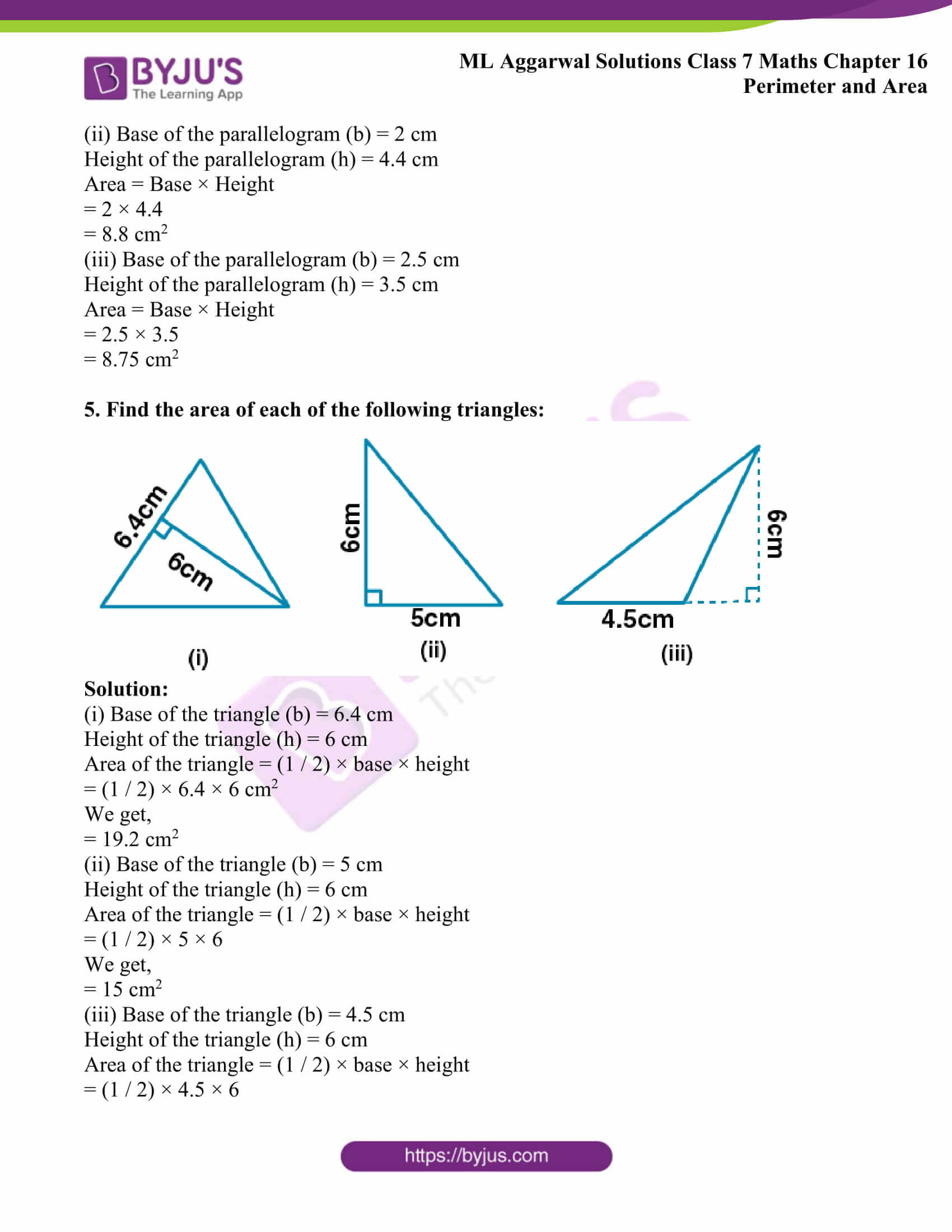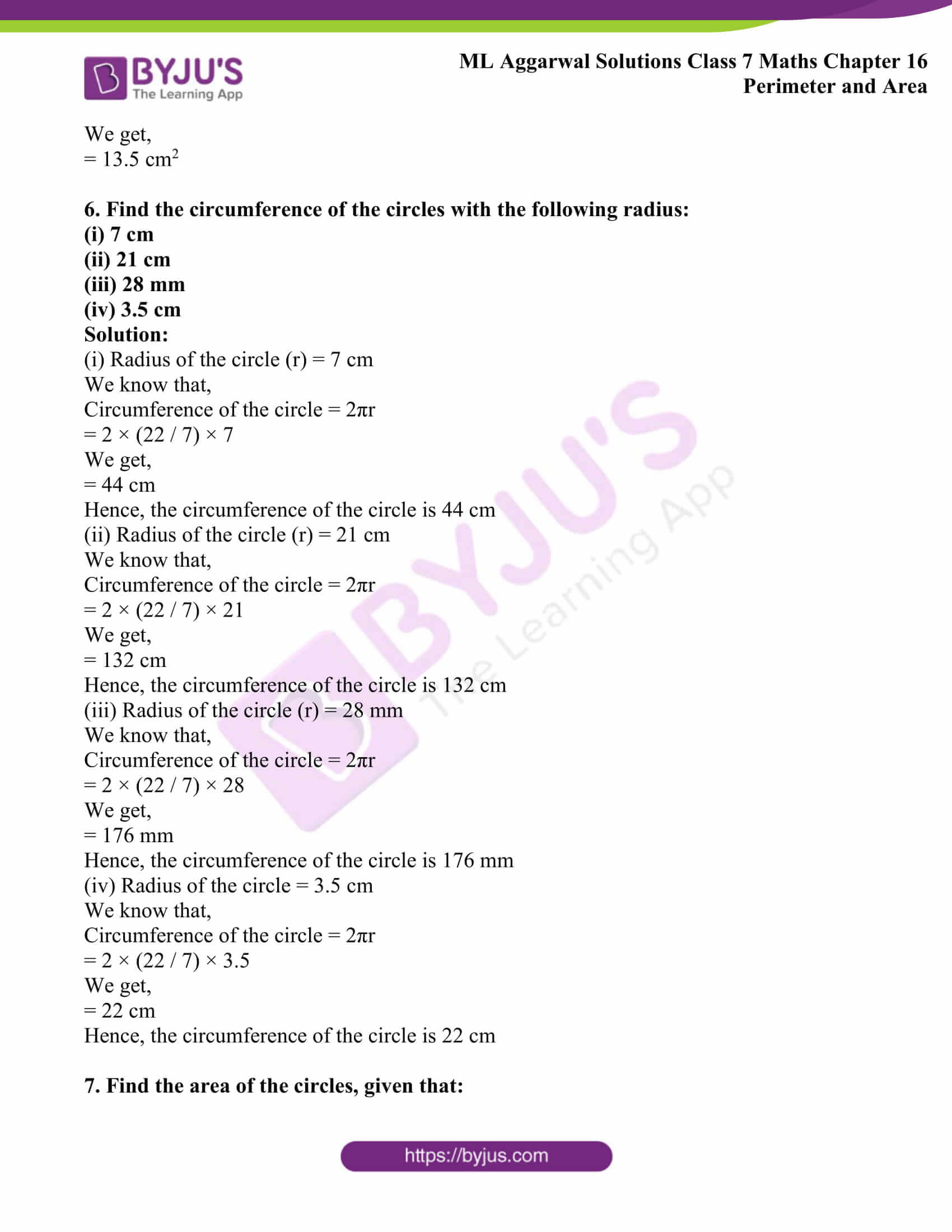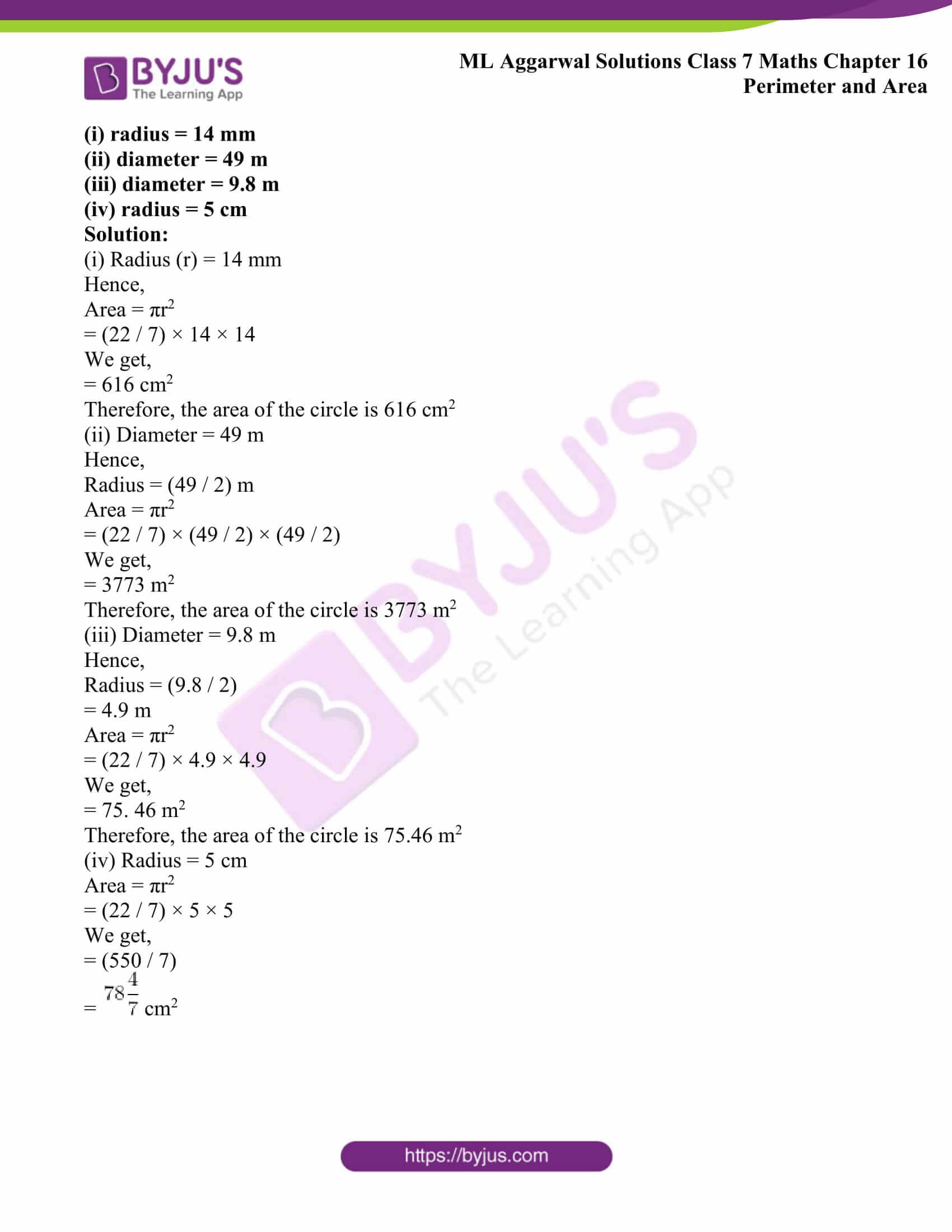## ML Aggarwal Solutions for Class 7 Maths Chapter 16 Perimeter and Area

1. ABCD is a square of side 24 cm. EF is parallel to BC and AE = 15 cm. By how much does

(i) the perimeter of AEFD exceed the perimeter of EBCF?

(ii) the area of AEFD exceed the area of EBCF?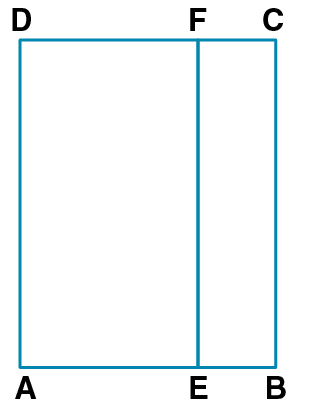Solution:

Given

The side of the square ABCD = 24 cm

AE is of length 15 cm

EB = 24 – 15

EB = 9 cm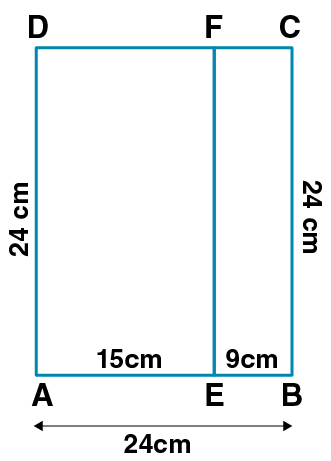(i) Perimeter of AEFD = 2 (15 + 24) cm

= 2 (39) cm

We get,

= 78 cm

Perimeter of EBCF = 2 (9 + 24) cm

= 2 (33) cm

We get,

= 66 cm

Now,

Difference between the two perimeters = 78 – 66

= 12 cm

(ii) Area of AEFD = l × b

= 15 × 24

We get,

= 360 sq. cm

Area of EBCF = 9 × 24

= 216 sq. cm

Now, difference between the two areas = 360 – 216

= 144 sq. cm

2. Nagma runs around a rectangular park 180 m long and 120 m wide at the rate of 7.5 km/ hour. In how much time will she complete five rounds?

Solution:

Length of rectangular plot (l) = 180 m

Breadth of rectangular (b) = 120 m

Perimeter = 2 (l + b)

= 2 (180 + 120) m

= 2 (300)

We get,

= 600 m

Distance travelled in 5 rounds = 600 × 5

= 3000 m i.e

= 3 km

Given speed = 7.5 km/hr

Time taken = (3 / 7.5) h

= {(3 × 10) / 75} h

= (30 / 75) h

We get,

= (2 / 5) h

= (2 / 5) × 60

= 2 × 12

= 24 minutes

3. The area of a rectangular plot is 540 m2, if its length is 27 m, find its breadth and perimeter.

Solution:

Given

Area of a rectangular plot = 540 m2

Length (l) = 27 m

Therefore,

= (540 / 27) m

We get,

= 20 m

And Perimeter = 2 (l + b)

= 2 (27 + 20) m

= 2 (47) m

We get,

= 94 m

Therefore, the breadth of the rectangular plot is 20 m and its perimeter is 94 m

4. Find the area of each of the following parallelogram: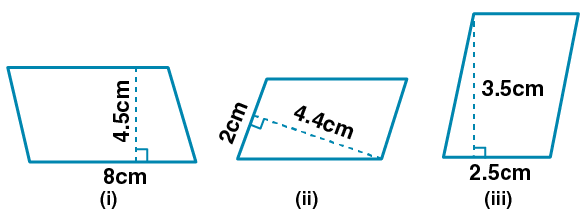Solution:

(i) Base of the parallelogram (b) = 8 cm and

Height of the parallelogram (h) = 4.5 cm

Area = Base × Height

= 8 × 4.5

= 36 cm2

(ii) Base of the parallelogram (b) = 2 cm

Height of the parallelogram (h) = 4.4 cm

Area = Base × Height

= 2 × 4.4

= 8.8 cm2

(iii) Base of the parallelogram (b) = 2.5 cm

Height of the parallelogram (h) = 3.5 cm

Area = Base × Height

= 2.5 × 3.5

= 8.75 cm2

5. Find the area of each of the following triangles: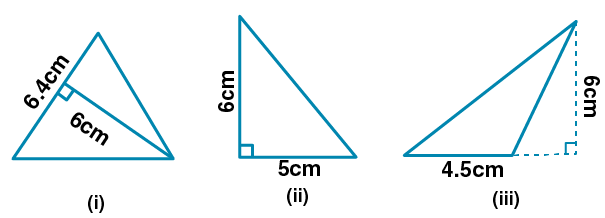Solution:

(i) Base of the triangle (b) = 6.4 cm

Height of the triangle (h) = 6 cm

Area of the triangle = (1 / 2) × base × height

= (1 / 2) × 6.4 × 6 cm2

We get,

= 19.2 cm2

(ii) Base of the triangle (b) = 5 cm

Height of the triangle (h) = 6 cm

Area of the triangle = (1 / 2) × base × height

= (1 / 2) × 5 × 6

We get,

= 15 cm2

(iii) Base of the triangle (b) = 4.5 cm

Height of the triangle (h) = 6 cm

Area of the triangle = (1 / 2) × base × height

= (1 / 2) × 4.5 × 6

We get,

= 13.5 cm2

6. Find the circumference of the circles with the following radius:

(i) 7 cm

(ii) 21 cm

(iii) 28 mm

(iv) 3.5 cm

Solution:

(i) Radius of the circle (r) = 7 cm

We know that,

Circumference of the circle = 2πr

= 2 × (22 / 7) × 7

We get,

= 44 cm

Hence, the circumference of the circle is 44 cm

(ii) Radius of the circle (r) = 21 cm

We know that,

Circumference of the circle = 2πr

= 2 × (22 / 7) × 21

We get,

= 132 cm

Hence, the circumference of the circle is 132 cm

(iii) Radius of the circle (r) = 28 mm

We know that,

Circumference of the circle = 2πr

= 2 × (22 / 7) × 28

We get,

= 176 mm

Hence, the circumference of the circle is 176 mm

(iv) Radius of the circle = 3.5 cm

We know that,

Circumference of the circle = 2πr

= 2 × (22 / 7) × 3.5

We get,

= 22 cm

Hence, the circumference of the circle is 22 cm

7. Find the area of the circles, given that:

(ii) diameter = 49 m

(iii) diameter = 9.8 m

Solution:

(i) Radius (r) = 14 mm

Hence,

Area = πr2

= (22 / 7) × 14 × 14

We get,

= 616 cm2

Therefore, the area of the circle is 616 cm2

(ii) Diameter = 49 m

Hence,

Radius = (49 / 2) m

Area = πr2

= (22 / 7) × (49 / 2) × (49 / 2)

We get,

= 3773 m2

Therefore, the area of the circle is 3773 m2

(iii) Diameter = 9.8 m

Hence,

= 4.9 m

Area = πr2

= (22 / 7) × 4.9 × 4.9

We get,

= 75. 46 m2

Therefore, the area of the circle is 75.46 m2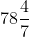cm2